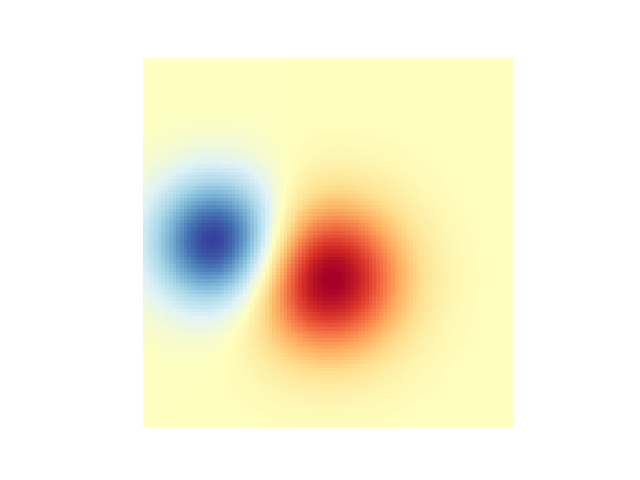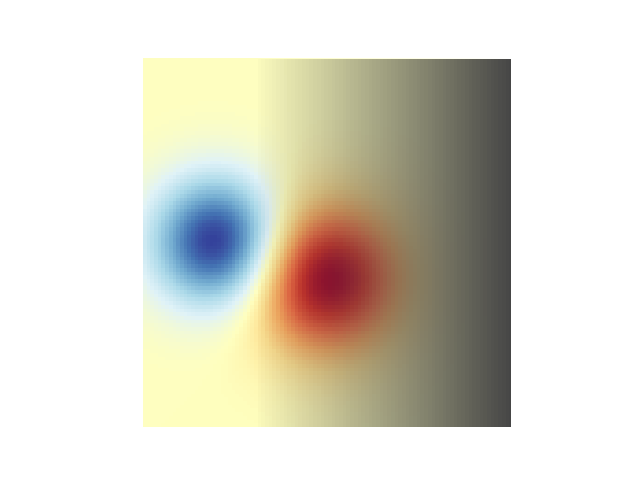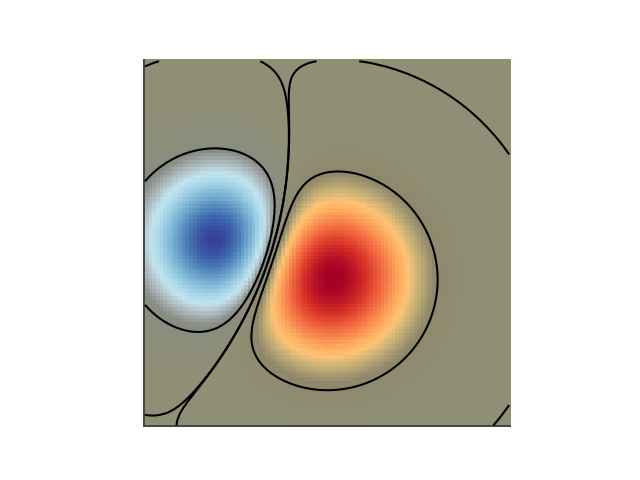# Blend transparency with color in 2D images#

Blend transparency with color to highlight parts of data with imshow.

A common use for matplotlib.pyplot.imshow is to plot a 2D statistical map. The function makes it easy to visualize a 2D matrix as an image and add transparency to the output. For example, one can plot a statistic (such as a t-statistic) and color the transparency of each pixel according to its p-value. This example demonstrates how you can achieve this effect.

First we will generate some data, in this case, we'll create two 2D "blobs" in a 2D grid. One blob will be positive, and the other negative.

import numpy as np
import matplotlib.pyplot as plt
from matplotlib.colors import Normalize

def normal_pdf(x, mean, var):
return np.exp(-(x - mean)**2 / (2*var))

# Generate the space in which the blobs will live
xmin, xmax, ymin, ymax = (0, 100, 0, 100)
n_bins = 100
xx = np.linspace(xmin, xmax, n_bins)
yy = np.linspace(ymin, ymax, n_bins)

# Generate the blobs. The range of the values is roughly -.0002 to .0002
means_high = [20, 50]
means_low = [50, 60]
var = [150, 200]

gauss_x_high = normal_pdf(xx, means_high, var)
gauss_y_high = normal_pdf(yy, means_high, var)

gauss_x_low = normal_pdf(xx, means_low, var)
gauss_y_low = normal_pdf(yy, means_low, var)

weights = (np.outer(gauss_y_high, gauss_x_high)
- np.outer(gauss_y_low, gauss_x_low))

# We'll also create a grey background into which the pixels will fade
greys = np.full((*weights.shape, 3), 70, dtype=np.uint8)

# First we'll plot these blobs using imshow without transparency.
vmax = np.abs(weights).max()
imshow_kwargs = {
'vmax': vmax,
'vmin': -vmax,
'cmap': 'RdYlBu',
'extent': (xmin, xmax, ymin, ymax),
}

fig, ax = plt.subplots()
ax.imshow(greys)
ax.imshow(weights, **imshow_kwargs)
ax.set_axis_off()## Blending in transparency#

The simplest way to include transparency when plotting data with matplotlib.pyplot.imshow is to pass an array matching the shape of the data to the alpha argument. For example, we'll create a gradient moving from left to right below.

# Create an alpha channel of linearly increasing values moving to the right.
alphas = np.ones(weights.shape)
alphas[:, 30:] = np.linspace(1, 0, 70)

# Create the figure and image
# Note that the absolute values may be slightly different
fig, ax = plt.subplots()
ax.imshow(greys)
ax.imshow(weights, alpha=alphas, **imshow_kwargs)
ax.set_axis_off()## Using transparency to highlight values with high amplitude#

Finally, we'll recreate the same plot, but this time we'll use transparency to highlight the extreme values in the data. This is often used to highlight data points with smaller p-values. We'll also add in contour lines to highlight the image values.

# Create an alpha channel based on weight values
# Any value whose absolute value is > .0001 will have zero transparency
alphas = Normalize(0, .3, clip=True)(np.abs(weights))
alphas = np.clip(alphas, .4, 1)  # alpha value clipped at the bottom at .4

# Create the figure and image
# Note that the absolute values may be slightly different
fig, ax = plt.subplots()
ax.imshow(greys)
ax.imshow(weights, alpha=alphas, **imshow_kwargs)

# Add contour lines to further highlight different levels.
ax.contour(weights[::-1], levels=[-.1, .1], colors='k', linestyles='-')
ax.set_axis_off()
plt.show()

ax.contour(weights[::-1], levels=[-.0001, .0001], colors='k', linestyles='-')
ax.set_axis_off()
plt.show()References

The use of the following functions, methods, classes and modules is shown in this example:

Keywords: matplotlib code example, codex, python plot, pyplot Gallery generated by Sphinx-Gallery